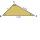Complementary angles 2

Two complementary angles are (x+4) and (2x - 7) find the value of x

Result

x =  31

Solution:

(x+4) + (2x - 7) = 90

3x = 93

x = 31

Calculated by our simple equation calculator.

Leave us a comment of example and its solution (i.e. if it is still somewhat unclear...):Be the first to comment!To solve this example are needed these knowledge from mathematics:

Do you have a linear equation or system of equations and looking for its solution? Or do you have quadratic equation? See also our right triangle calculator. See also our trigonometric triangle calculator.

Next similar examples:

1. Find the 9Find the missing angle in the triangle and then name triangle. Angles are: 95, 2x+15, x+3
2. Internal anglesOne internal angle of the triangle JAR is 25 degrees. The difference is the size of the two other is 15°. Identify the size of these angles.
3. Plane IIA plane flew 50 km on a bearing 63degrees20 and the flew on a bearing 153degrees20 for 140km. Find the distance between the starting point and the ending point
4. Type of triangleHow do I find the triangle type if the angle ratio is 2:3:7 ?
5. DiagonalCan a rhombus have the same length diagonal and side?The ladder has a length 3.5 meters. He is leaning against the wall so that his bottom end is 2 meters away from the wall. Determine the height of the ladder.
7. Broken treeThe tree is broken at 4 meters above the ground and the top of the tree touches the ground at a distance of 5 from the trunk. Calculate the original height of the tree.
8. Height 2Calculate the height of the equilateral triangle with side 38.
9. Obtuse angleWhich obtuse angle is creating clocks at 17:00?
10. Center traverseIt is true that the middle traverse bisects the triangle?
11. EquationSolve the equation: 1/2-2/8 = 1/10; Write the result as a decimal number.
12. Unknown numberIdentify unknown number which 1/5 is 40 greater than one tenth of that number.
13. Simple equationSolve for x: 3(x + 2) = x - 18
14. Equation with xSolve the following equation: 2x- (8x + 1) - (x + 2) / 5 = 9
15. Percentage - fractionsAbout what percentage we must increase number 1/6 to get number 1/3?
16. GardenThe garden around the new majer is divided as follows: 35% vegetable, 30% fruit orchards, 10% flowers and the remaining 120 m2 are lawns. What is the total area of the garden?
17. 1.5 divided1.5 divided by 1 = w divided by 4